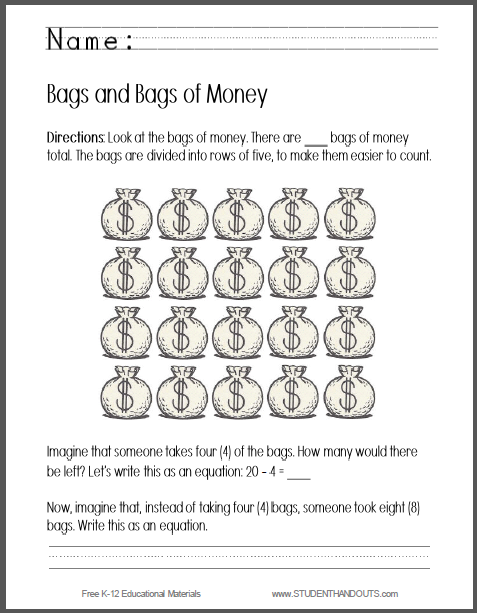Bags of Money - Writing an Equation Worksheet | Student Handouts
 Share the learning joy!

Bags of Money Worksheet - Writing an EquationDirections: 1 Look at the bags of money. There are _____ bags of money total. The bags are divided into rows of five, to make them easier to count. 2 Imagine that someone takes four (4) of the bags. How many would there be left? Let's write this as an equation: 20 - 4 = _____ 3 Imagine that someone takes four (4) of the bags. How many would there be left? Let's write this as an equation: 20 - 4 = _____ 4 Now, imagine that, instead of taking four (4) bags, someone took eight (8) bags. Write this as an equation. Click here to print this worksheet.

CCSS.MATH.CONTENT.K.OA.A.2 - Solve addition and subtraction word problems, and add and subtract within 10, e.g., by using objects or drawings to represent the problem.